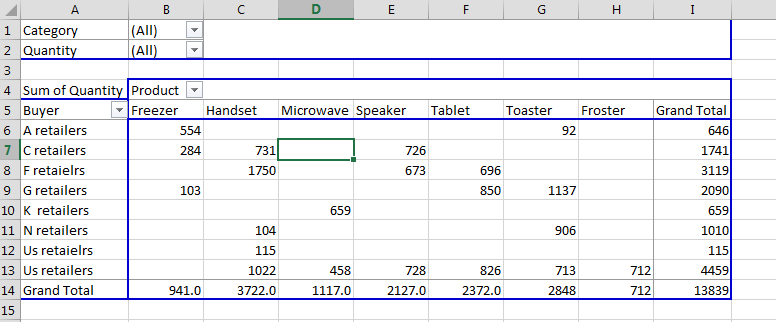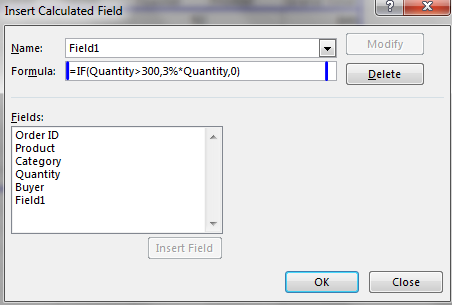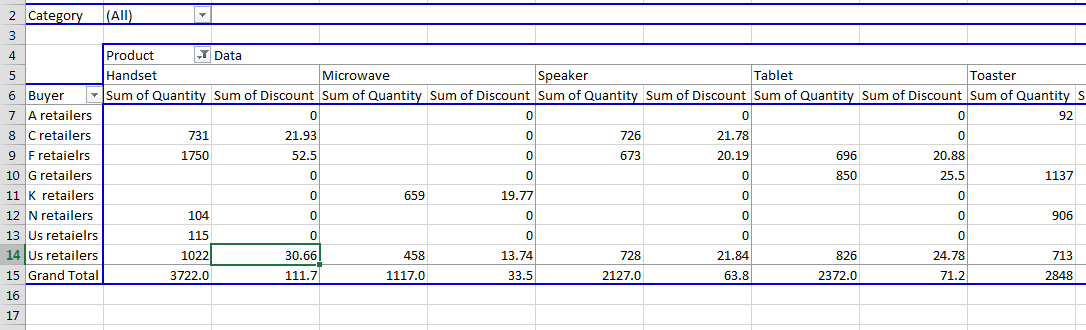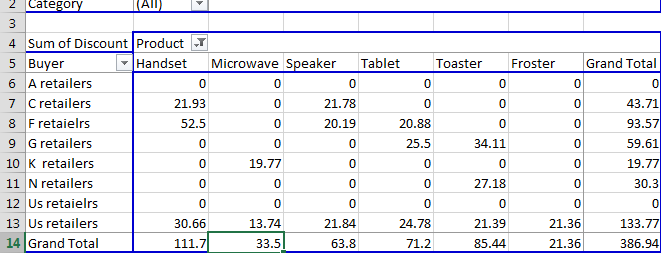#### Modifying a calculated field in a pivot table

In this lesson you will learn how to modify a calculated field in your pivot table. You need this to make proper calculation which you need at the moment.

## Pivot table data preparation

Open sheet with your pivot table and click any cell in the pivot table to get it activated.

##Adding a calculated field to pivot table

Go to Analyze tab. Select Fields, Items and Sets button and click Calculated Field.

Enter the formula as per your requirements.Excel will display the pivot table with an additional column (sum of discount in this case).Click anywhere in the pivot table. PivotTable Fields appears on the right. Under sum of values, go to sum of quantity and click remove field.### Modifying a calculated field in pivot table

As you see you have Sum of Discount instead of Sum of Quantity. Now you know how to modify calculated field in Pivot Tables. Use this to make your customized calculations.

## Using Calculated Fields to Create Custom Reports

Calculated fields can be used to create custom reports in Excel. This can be useful for businesses that need to track specific data or trends that are not available in the standard pivot table reports.

For example, the following calculated field can be used to create a report that shows the total sales for each product category, as well as the percentage of total sales for each product category:

`=SUM(Sales) / SUM(Sales)`

This formula works by first calculating the total sales for each product category. Then, it divides the total sales for each product category by the total sales for all product categories. This gives you the percentage of total sales for each product category.